# Divisibility by 2, 5 and 10

Divisibility by 2, 5 and 10#### Rule for Divisibility by 2#### Rule for Divisibility by 5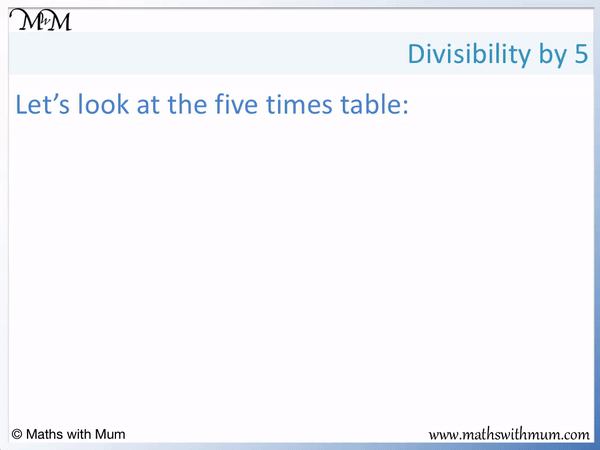#### Rule for Divisibility by 10• If a number is divisible by 2, it means that it is in the 2 times table.
• If a number is divisible by 5 or 10, it means that it is in either the 5 or 10 times table respectively.
• To check if a number is divisible by 2, 5 and 10, look at its final digit.
• A number is divisible by 2 if it ends in 2, 4, 6, 8 or 0.
• A number is divisible by 5 if it ends in 5 or 0.
• A number is divisible by 10 if it ends in a 0.
To test for divisibility by 2, 5 or 10, look at the final digit of the number.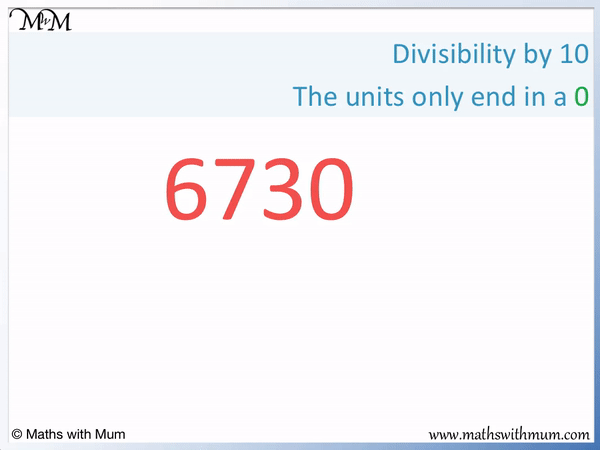• The last digit of the number 6730 is 0.
• A number is divisible by 2 if it ends in 2, 4, 6, 8 or 0.
• The number ends in a 0 and so it is divisible by 2.
• A number is divisible by 5 if it ends in 5 or 0.
• The number ends in a 0 and so it is divisible by 5.
• A number is divisible by 10 if it ends in a 0.
• The number ends in a 0 and so it is divisible by 10.
• A number that ends in 0 is divisible by 2, 5 and 10.
• 6730 is divisible by 2, 5 and 10, which means that it is in the 2, 5 and 10 times table.# Divsibility by 2, 5 and 10

## How to Check Divisibility by 2, 5 or 10

To check if a number is divisible by 2, 5 or 10, follow these steps:

• Look at the last digit of the number.
• If the digit is a 2, 4, 6, 8 or 0, the number is divisible by 2.
• If the digit is a 5 or a 0, the number is divisible by 5.
• If the digit is a 0, the number is divisible by 10.

For example, here is 68. We will check if it is divisible by 2, 5 or 10.

Its last digit is 8.

If the digit is a 2, 4, 6, 8 or 0, the number is divisible by 2. Therefore 68 is divisible by 2.The last digit must be a 5 or a 0 for the number to be divisible by 5. 8 is neither of these digits and so, 68 is not divisible by 5. It is not in the 5 times table. A number can be divisible by 2, 5 and 10. If a number is divisible by 10, it is also divisible by 2 and 5.

For example, here is 70.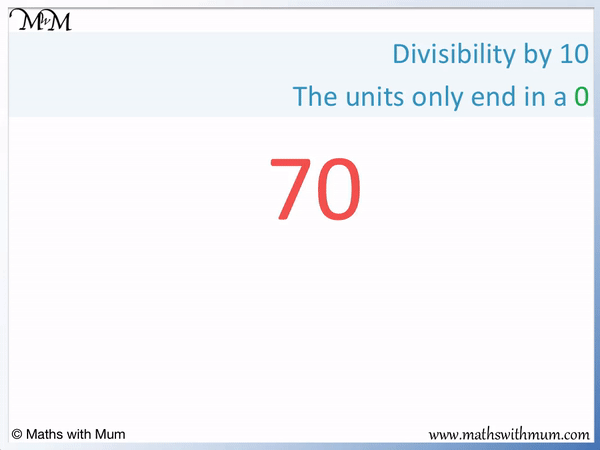70 ends with a digit of 0.

A number must end in a 0 to be divisible by 10. 70 is in the 10 times table. It is divisible by 10.

If the digit is a 2, 4, 6, 8 or 0, the number is divisible by 2. Since 70 has a final digit of 0, it is divisible by 2.

A number must end in a 5 or a 0 to be divisible by 5. 70 ends in a 0 and so, it is divisible by 5 too.

A number divisible by 10 is also divisible by 2 and 5 because 2 and 5 both divide into 10.

## Which of the Following Numbers are Divisible by 2, 5 or 10?

If a number ends in 2, 4, 6, 8 or 0, it is divisible by 2. If it ends in 5 or 0, it is divisible by 5. If it ends in 0, it is divisible by 10. If it is divisible by 10, it is also divisible by 2 and 5.

Here is a table of examples of numbers that will be checked for divisibility by 2, 5 and 10 using this rule.

Number Last Digit Divisible by 2 Divisible by 5 Divisible by 10
42 2
25 5
80 0
1908 8
7540 0
3895 5

42 ends in a 2 and so, it is divisible by 2 but not 5 or 10.

25 ends in a 5 and so, it is divisible by 5 but not 2 or 10.

80 ends in a 0 and so, it is divisible by 2, 5 and 10.

1908 ends in an 8 and so, it is divisible by 2 but not 5 or 10.

7540 ends in a 0 and so, it is divisible by 2, 5 and 10.

3895 ends in a 5 and so, it is divisible by 5 but not 2 or 10.

## Rule for Divisibilty by 2

A number is divisible by 2 only if its last digit is a 2, 4, 6, 8 or 0. For example, 426 is divisible by 2 because it ends in a 2. 327 is not divisible by 2 because it ends in a 7.

If a number is divisible by 2, it means that is can be divided by 2 exactly without a remainder. It is in the 2 times table.

All numbers in the 2 times table repeat the pattern of the last digit ending in 2, 4, 6, 8 and 0.Every time we get to a number ending in 0, the pattern starts again.

This means that to check if a number is a multiple of 2, simply look at the last digit and ignore all preceding digits. If the number ends in 2, 4, 6, 8 or 0, the number is a multiple of 2.

For example, we will use the rule to test if 68 is divisible by 2.68 ends in an 8, which is one of the digits 2, 4, 6, 8 or 0.

68 is divisible by 2 because it is an even number.

It does not matter how large the number is, only look at the last digit to decide if a number is divisible by 2. For example, here is 37,110.37,100 ends in a 0. 0 is an even number and so, 37,100 is divisible by 2.

Here is 209, which ends in a 9.A number must end in 2, 4, 6, 8 or 0 to be divisible by 2. If a number ends in 9 it is not divisible by 2.

209 is not divisible by 2.

## Rule for Divisibilty by 5

A number is divisible by 5 only if its last digit is a 5 or a 0. For example, 935 is divisible by 5 because it ends in a 5. 732 is not divisible by 5 because it ends in a 2.

If a number is divisible by 5, this means it can be divided exactly by 5 with no remainder. It is in the 5 times table.

All numbers in the 5 times table repeat the pattern of ending in 5 or 0.If a number ends in any other digit apart from 5 or 0, it is not in the 5 times table.

For example, here is 45.45 ends in a 5. Numbers that end in 5 or 0 are divisible by 5 and so, 45 is divisible by 5.

It does not matter how large a number is. If a number ends in a 5 or a 0, it is in the 5 times table. We only need to look at the last digit.

Here is 17,760.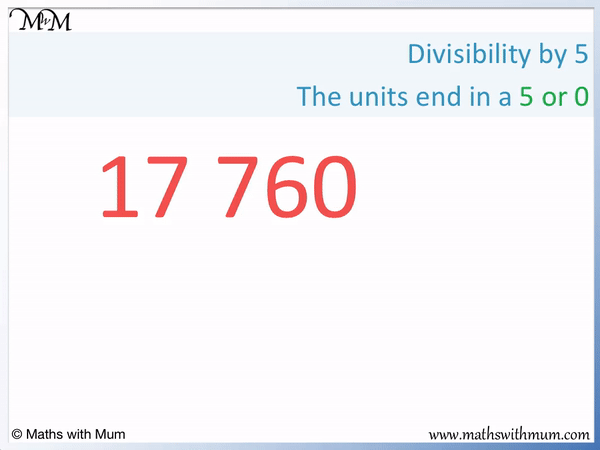17,760 ends in a 0. If a number ends in a 5 or a 0, it is divisible by 5.

Therefore 17,760 is divisible by 5.

Here is 803.803 ends in a 3. To be divisible by 5, a number can only end in a 5 or a 0.

803 is not divisible by 5.

## Rule for Divisibilty by 10

A number is divisible by 10 only if it ends in a 0. For example, 770 is divisible by 10 because it ends in a 0. 565 is not divisible by 10 because it ends in a 5.

If a number is divisible by 10, this means that is can be divided exactly by 10 with no remainder. It is in the 10 times table.

All numbers in the 10 times table end in a 0.

If a number does not end in a 0, it is not in the 10 times table.For example, here is 70.70 ends in a 0. If a number ends in a 0, it is in the 10 times table and can be divided exactly by 10.

70 is divisible by 10.

It does not matter how large a number is, if it ends in a 0, it is divisible by 10.

For example, here is 2,070,420.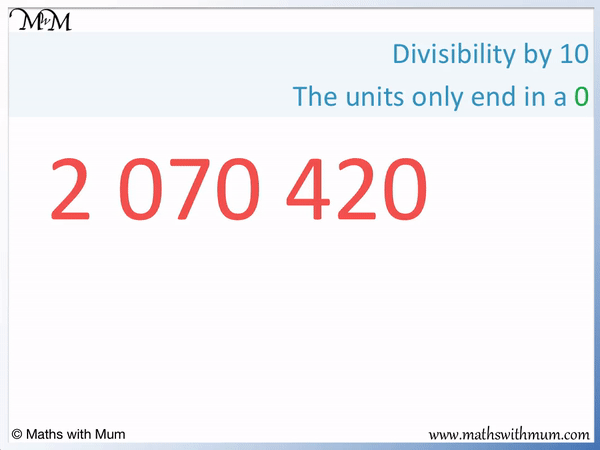2,070,420 ends in a 0. We do not need to look at the digits in front of the last digit to decide.

2,070,420 is divisible by 10 because it ends in a 0.

Here is the example of 177.177 ends in a 7. For a number to be divisible by 10, it must end in a 0.

177 is not divisible by 10.Now try our lesson on Divisibility by Four where we learn how to check if a number is divisible by 4.error: Content is protected !!